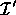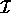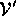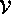Next: Octree Subdivision Up: Performance and Optimization Previous: Performance and Optimization

## Piecewise Linear ApproximationFigure 4: 2D analogue of piecewise linear warping. A warped imageis first subdivided by an adaptive grid of squares, here marked by solid lines. Then, each square vertex is warped into. Finally, pixels in the interior of each grid cell are warped by bilinearly interpolating the warped positions of the vertices. The dashed arrows demonstrate how the interior of the bottom right square is warped. The dotted rectangles mark image buffer borders.

The 2D form of this optimization, shown in figure 4, illustrates its key steps within the familiar framework of image warping. In 3D, piecewise linear warping begins by subdividinginto a coarse, 3D, regular grid, and warping the grid vertices into, using the algorithm of section 3.1. The voxels in the interior of each cubic grid cell are then warped by trilinearly interpolating the warped positions of the cube's vertices. Using this method,can be computed by scan-converting each cube in turn. Essentially, we treatas a solid texture, with the warped grid specifying the mapping into texture space. The expensive computation of section 3.1 is now performed only for a small fraction of the voxels, and scan-conversion dominates the warping time.

This piecewise linear approximation will not accurately capture the warp in highly non-linear regions, unless we use a very fine grid. However, computing a uniformly fine sampling of the warp defeats the efficiency gain of this approach. Hence, we use an adaptive grid which is subdivided more finely in regions where the warp is highly non-linear. To determine whether a grid cell requires subdivision, we compare the exact and approximated warped positions of several points within the cell. If the error is above a user-specified threshold, the cell is subdivided further. In order to reduce computation, we use the vertices of the next-higher resolution grid as the points at which to measure the error. Using this technique, the non-linear warp can be approximated to arbitrary accuracy.Since we are subsampling the warping function, it is possible that this algorithm will fail to subdivide non-linear regions. Analytically bounding the variance of the warping function would guarantee conservative subdivision. However, this is unnecessary in practice, as the warps used in generating morphs generally do not possess large high-frequency components.

This optimization has been applied to 2D morphing systems, as well; by using common texture-mapping hardware to warp the images, 2D morphs can be generated at interactive rates .Next: Octree Subdivision Up: Performance and Optimization Previous: Performance and Optimization

Last update: 11 May 1995 by Apostolos "Toli" Lerios
tolis@cs.stanford.edu